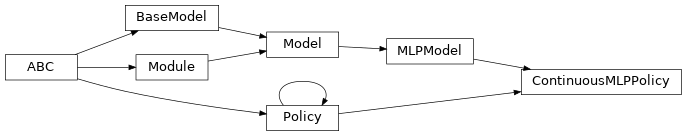# garage.tf.policies.continuous_mlp_policy¶

This modules creates a continuous MLP policy network.

A continuous MLP network can be used as policy method in different RL algorithms. It accepts an observation of the environment and predicts a continuous action.

class ContinuousMLPPolicy(env_spec, name='ContinuousMLPPolicy', hidden_sizes=(64, 64), hidden_nonlinearity=tf.nn.relu, hidden_w_init=tf.initializers.glorot_uniform(seed=deterministic.get_tf_seed_stream()), hidden_b_init=tf.zeros_initializer(), output_nonlinearity=tf.nn.tanh, output_w_init=tf.initializers.glorot_uniform(seed=deterministic.get_tf_seed_stream()), output_b_init=tf.zeros_initializer(), layer_normalization=False)Continuous MLP Policy Network.

The policy network selects action based on the state of the environment. It uses neural nets to fit the function of pi(s).

Parameters
• env_spec (garage.envs.env_spec.EnvSpec) – Environment specification.

• name (str) – Policy name, also the variable scope.

• hidden_sizes (list[int]) – Output dimension of dense layer(s). For example, (32, 32) means the MLP of this policy consists of two hidden layers, each with 32 hidden units.

• hidden_nonlinearity (callable) – Activation function for intermediate dense layer(s). It should return a tf.Tensor. Set it to None to maintain a linear activation.

• hidden_w_init (callable) – Initializer function for the weight of intermediate dense layer(s). The function should return a tf.Tensor.

• hidden_b_init (callable) – Initializer function for the bias of intermediate dense layer(s). The function should return a tf.Tensor.

• output_nonlinearity (callable) – Activation function for output dense layer. It should return a tf.Tensor. Set it to None to maintain a linear activation.

• output_w_init (callable) – Initializer function for the weight of output dense layer(s). The function should return a tf.Tensor.

• output_b_init (callable) – Initializer function for the bias of output dense layer(s). The function should return a tf.Tensor.

• layer_normalization (bool) – Bool for using layer normalization or not.

build(self, obs_var, name=None)

Symbolic graph of the action.

Parameters
• obs_var (tf.Tensor) – Tensor input for symbolic graph.

• name (str) – Name for symbolic graph.

Returns

symbolic graph of the action.

Return type

tf.Tensor

property input_dim(self)

int: Dimension of the policy input.

get_action(self, observation)

Get single action from this policy for the input observation.

Parameters

observation (numpy.ndarray) – Observation from environment.

Returns

Predicted action. dict: Empty dict since this policy does not model a distribution.

Return type

numpy.ndarray

get_actions(self, observations)

Get multiple actions from this policy for the input observations.

Parameters

observations (numpy.ndarray) – Observations from environment.

Returns

Predicted actions. dict: Empty dict since this policy does not model a distribution.

Return type

numpy.ndarray

get_regularizable_vars(self)

Get regularizable weight variables under the Policy scope.

Returns

List of regularizable variables.

Return type

list(tf.Variable)

property env_spec(self)

Policy environment specification.

Returns

Environment specification.

Return type

garage.EnvSpec

clone(self, name)

Return a clone of the policy.

It copies the configuration of the primitive and also the parameters.

Parameters

name (str) – Name of the newly created policy.

Returns

Clone of this object

Return type

garage.tf.policies.ContinuousMLPPolicy

network_input_spec(self)

Network input spec.

Returns

List of key(str) for the network inputs.

Return type

list[str]

network_output_spec(self)

Network output spec.

Returns

List of key(str) for the network outputs.

Return type

list[str]

property parameters(self)

Parameters of the model.

Returns

Parameters

Return type

np.ndarray

property name(self)

Name (str) of the model.

This is also the variable scope of the model.

Returns

Name of the model.

Return type

str

property input(self)

Default input of the model.

When the model is built the first time, by default it creates the ‘default’ network. This property creates a reference to the input of the network.

Returns

Default input of the model.

Return type

tf.Tensor

property output(self)

Default output of the model.

When the model is built the first time, by default it creates the ‘default’ network. This property creates a reference to the output of the network.

Returns

Default output of the model.

Return type

tf.Tensor

property inputs(self)

Default inputs of the model.

When the model is built the first time, by default it creates the ‘default’ network. This property creates a reference to the inputs of the network.

Returns

Default inputs of the model.

Return type

list[tf.Tensor]

property outputs(self)

Default outputs of the model.

When the model is built the first time, by default it creates the ‘default’ network. This property creates a reference to the outputs of the network.

Returns

Default outputs of the model.

Return type

list[tf.Tensor]

reset(self, do_resets=None)

Reset the module.

This is effective only to recurrent modules. do_resets is effective only to vectoried modules.

For a vectorized modules, do_resets is an array of boolean indicating which internal states to be reset. The length of do_resets should be equal to the length of inputs.

Parameters

do_resets (numpy.ndarray) – Bool array indicating which states to be reset.

property state_info_specs(self)

State info specification.

Returns

keys and shapes for the information related to the

module’s state when taking an action.

Return type

List[str]

property state_info_keys(self)

State info keys.

Returns

keys for the information related to the module’s state

when taking an input.

Return type

List[str]

terminate(self)

Clean up operation.

get_trainable_vars(self)

Get trainable variables.

Returns

A list of trainable variables in the current

variable scope.

Return type

List[tf.Variable]

get_global_vars(self)

Get global variables.

Returns

A list of global variables in the current

variable scope.

Return type

List[tf.Variable]

get_params(self)

Get the trainable variables.

Returns

A list of trainable variables in the current

variable scope.

Return type

List[tf.Variable]

get_param_shapes(self)

Get parameter shapes.

Returns

A list of variable shapes.

Return type

List[tuple]

get_param_values(self)

Get param values.

Returns

Values of the parameters evaluated in

the current session

Return type

np.ndarray

set_param_values(self, param_values)

Set param values.

Parameters

param_values (np.ndarray) – A numpy array of parameter values.

flat_to_params(self, flattened_params)

Unflatten tensors according to their respective shapes.

Parameters

flattened_params (np.ndarray) – A numpy array of flattened params.

Returns

A list of parameters reshaped to the

shapes specified.

Return type

List[np.ndarray]

property observation_space(self)

Observation space.

Returns

The observation space of the environment.

Return type

akro.Space

property action_space(self)

Action space.

Returns

The action space of the environment.

Return type

akro.Space